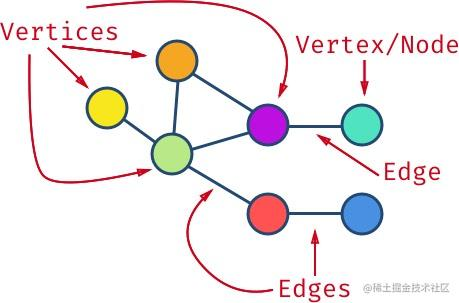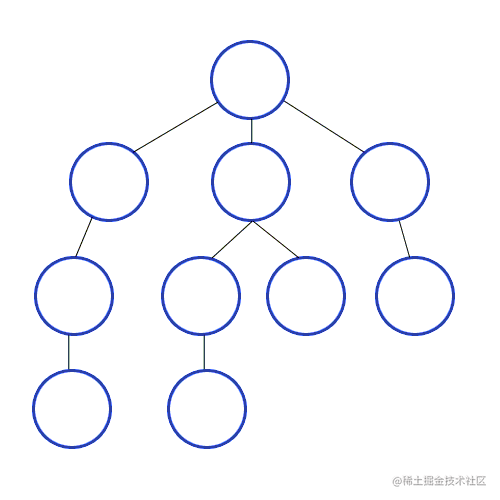# [译文] 初学者应该了解的数据结构： Graph

1. 算法的时间复杂性与大 O 符号
2. 每个程序员应该知道的八种时间复杂度
3. 初学者应该了解的数据结构：Array、HashMap 与 List (译文)
4. 初学者应该了解的数据结构： Graph 👈 即本文
5. 初学者应该了解的数据结构：Tree (敬请期待)
6. 附录 I：递归算法分析

# 图的基础Graph is composed of vertices and edges

# 图的应用

• 航空线路图 (如上图所示)

• 顶点 = 机场
• 边 = 两个机场间的飞行线路
• 权重 = 两个机场间的距离
• GPS 导航

• 顶点 = 交叉路口
• 边 = 道路
• 权重 = 从一个路口到另一个路口所花的时间
• 网络

• 顶点 = 服务器
• 边 = 数据链路
• 权重 = 连接速度

• 电子电路
• 航空控制
• 行车导航
• 电信设施： 基站建设规划
• 社交网络： Facebook 利用图来推荐（你可能认识的）朋友
• 推荐系统： Amazon/Netflix 利用图来推荐产品与电影
• 利用图来规划物流线路

# 图的表示

1. 邻接表
2. 邻接矩阵

## 邻接矩阵

``````  a b c d e
a 1 1 - - -
b - - 1 - -
c - - - 1 -
d - 1 1 - -

（因此，）在邻接矩阵中添加一个顶点的时间复杂度是 O(|V|²)

``````  a b c d e
b - - 1 - -

## 邻接表

``````a -> { a b }
b -> { c }
c -> { d }
d -> { b c }

# 基于 HashMap 实现的邻接表

``````const graph = {
a：[ 'a'，'b' ]，
b：[ 'c' ]，
c：[ 'd' ]，
d：[ 'b'，'c' ]
};

• 添加或删除顶点。
• 添加或删除边。

# 基于邻接表，以面向对象风格实现图

``````class Node {
constructor(value) {
this.value = value;
}

}

const index = this.adjacents.indexOf(node);
if (index > -1) {
return node;
}
}

}

return this.adjacents.indexOf(node) > -1;
}
}

Make it work. Make it right. Make it faster.

Graph.constructor

``````class Graph {
constructor(edgeDirection = Graph.DIRECTED) {
this.nodes = new Map();
this.edgeDirection = edgeDirection;
}
// ...
}
Graph.UNDIRECTED = Symbol('directed graph'); // one-way edges
Graph.DIRECTED = Symbol('undirected graph'); // two-ways edges

``````addEdge(source, destination) {
const sourceNode = this.addVertex(source);
const destinationNode = this.addVertex(destination);
if(this.edgeDirection === Graph.UNDIRECTED) {
}
return [sourceNode, destinationNode];
}

``````addVertex(value) {
if(this.nodes.has(value)) {
return this.nodes.get(value);
} else {
const vertex = new Node(value);
this.nodes.set(value, vertex);
return vertex;
}
}

Graph.removeVertex

``````removeVertex(value) {
const current = this.nodes.get(value);
if(current) {
for (const node of this.nodes.values()) {
}
}
return this.nodes.delete(value);
}

Graph.removeEdge

``````removeEdge(source, destination) {
const sourceNode = this.nodes.get(source);
const destinationNode = this.nodes.get(destination);
if(sourceNode && destinationNode) {
if(this.edgeDirection === Graph.UNDIRECTED) {
}
}
return [sourceNode, destinationNode];
}

• 如果边两端的顶点不存在，不再需要创建它。
• 使用`Node.removeAdjacent` 而不是 `Node.addAdjacent`

# 广度优先搜索(BFS) - 图的搜索

``````*bfs(first) {
const visited = new Map();
const visitList = new Queue();
while(!visitList.isEmpty()) {
const node = visitList.remove();
if(node && !visited.has(node)) {
yield node;
visited.set(node);
}
}
}

``````const graph = new Graph(Graph.UNDIRECTED);
const [first] = graph.addEdge(1, 2);
bfsFromFirst = graph.bfs(first);
bfsFromFirst.next().value.value; // 1
bfsFromFirst.next().value.value; // 2
bfsFromFirst.next().value.value; // 3
bfsFromFirst.next().value.value; // 4
// ...

# 深度优先搜索 (DFS) -图的搜索Depth First Search in a graph

DFS 的实现近似于 BFS，但使用的是栈而不是队列：

``````*dfs(first) {
const visited = new Map();
const visitList = new Stack();
while(!visitList.isEmpty()) {
const node = visitList.remove();
if(node && !visited.has(node)) {
yield node;
visited.set(node);
}
}
}

``````const graph = new Graph(Graph.UNDIRECTED);
const [first] = graph.addEdge(1, 2);
dfsFromFirst = graph.dfs(first);
visitedOrder = Array.from(dfsFromFirst);
const values = visitedOrder.map(node => node.value);
console.log(values); // [1, 4, 8, 3, 7, 6, 10, 2, 5, 9]